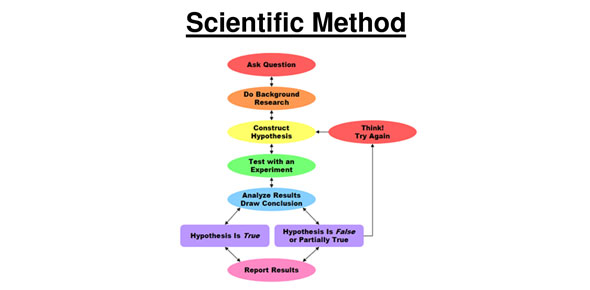# Scientific Method Review Quiz

15 Questions | Attempts: 894SettingsPractice quiz to review scientific method

• 1.
"What effect does temperature have on plant height?" is an example of a conclusion.
• A.

True

• B.

False

• 2.
The manipulated variable is purposely changed to test a hypothesis.
• A.

True

• B.

False

• 3.
Gram is the unit used to measure the mass of an object.
• A.

True

• B.

False

• 4.
The graduated cylinder is the most appropriate tool used to measure length.
• A.

True

• B.

False

• 5.
The inch is the most appropriate unit to measure the length of a swimming pool.
• A.

True

• B.

False

• 6.
The hypothesis is the curved surface of a liquid.
• A.

True

• B.

False

• 7.
This is used to solve questions/problems
• 8.
Data is displayed here to show patterns or trends or results from the experiment.
• 9.
Using one or more of your senses to gather information
• 10.
Prediction to, or possible explanation for, the scientific question.
• 11.
Part of the procedure that has no treatment, used to make comparisons
• 12.
Explain the difference between a manipulated variable and a responding variable.
• 13.
The  is purposely changed to test a hypothesis.
• 14.
is the metric unit used to measure mass
• 15.
Data is recorded in a  and displayed in a  .

## Related TopicsBack to top
×

Wait!
Here's an interesting quiz for you.